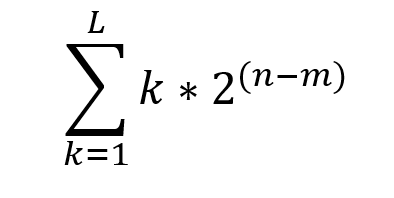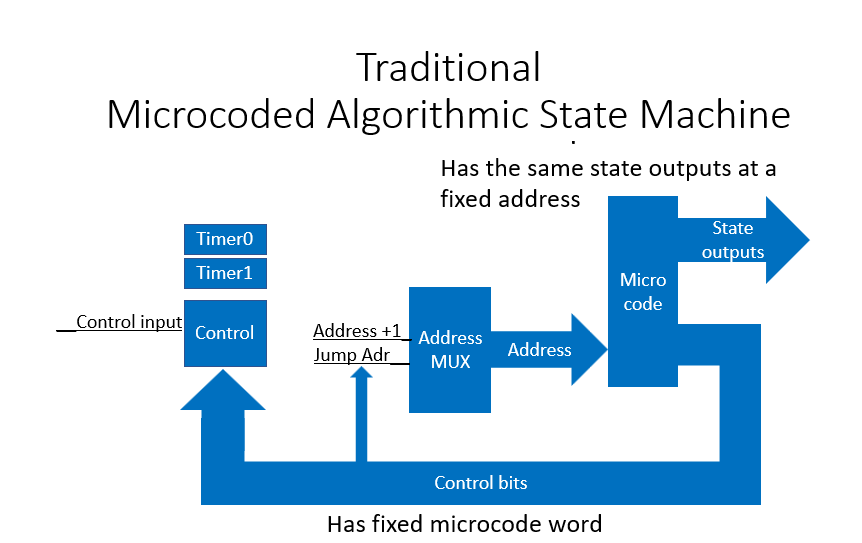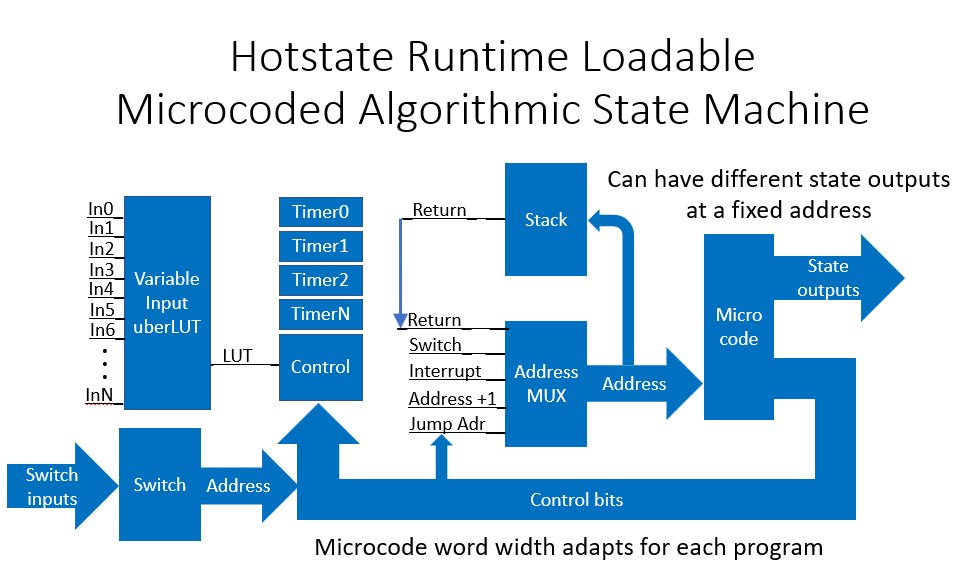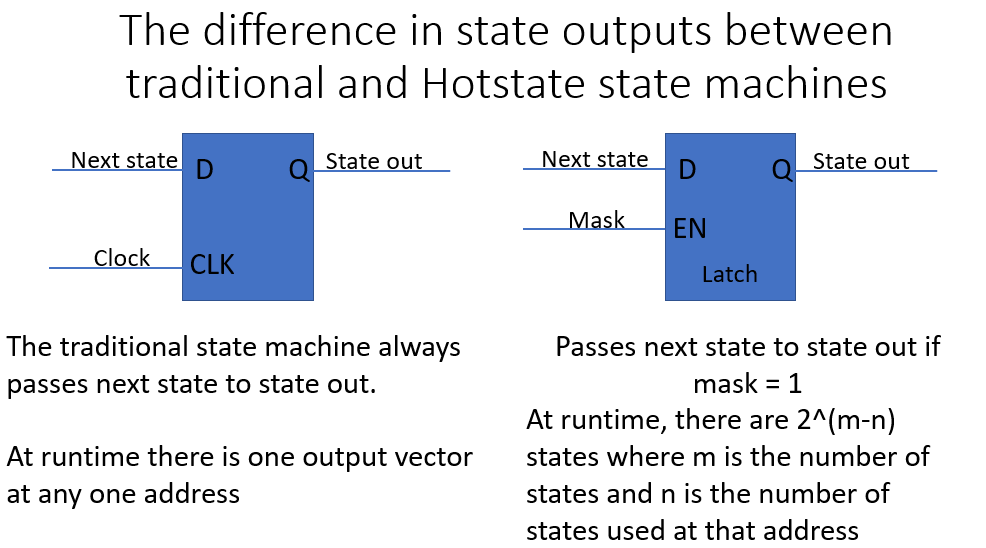A traditional state machine produces the same output state vector at the same address. At compile time it can have $2^n*L$possible states where n is the number of output states and L is the number of lines of microcode.

At runtime, the traditional state machine has L different state vectors.

Hotstate Outputs
To control state outputs stimulatingly use the comma operator.
LED0 = 1, LED2 = 0; // This is 100% C
These states will toggle at the same time leaving all other states quiescent.
The state outputs are qualified by the corresponding mask bit and latched if appropriate. The mask bit and state bits are combined into the state output.

At compile time the hotstate machines have $2^n*L$ possible states where n is the number of output states and L is the number of lines of microcode.

At runtime the possible number of state outputs at any one address during run time iswhere n is the total number of states and m is the number of states used in that line of code and L is the total number of lines of microcode.

In the traditional algorithmic state, there is one (1) state vector per address.